Updating search results...

# 40 Results

View
Selected filters:
• equalConditional Remix & Share Permitted
CC BY-NC
Rating
0.0 stars

Multi-day hands on lesson to teach strategies for comparing sets: matching, counting, equal shares

Subject:
Mathematics
Material Type:
Lesson Plan
Author:
HEATHER LANDRETH
07/30/2019Unrestricted Use
CC BY
Rating
0.0 stars

Multi-day hands on lesson to teach strategies for comparing sets: matching, counting, equal shares

Subject:
Mathematics
Material Type:
Activity/Lab
Lesson
Author:
SHAKEKIA MOSS
07/09/2020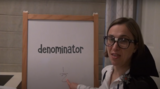Unrestricted Use
CC BY
Rating
0.0 stars

This lesson is Day 10 in a series of 12 lessons around fraction equivalences and comparisons.

This video lesson serves as a review of equpartitioning in order to determine the fractional part of an area or length. This is a necessary foundational skill for comparing and finding equivalent fractions.

Subject:
Mathematics
Material Type:
Activity/Lab
Interactive
Lesson
Simulation
Author:
Dawne Coker
06/25/2020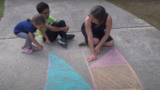Unrestricted Use
CC BY
Rating
0.0 stars

This lesson is Day 11 in a series of 12 lessons around fraction equivalences and comparisons.

This video lesson reviews parts of a whole, comparing fractions, and equivalent fractions. No materials are needed.

Subject:
Mathematics
Material Type:
Activity/Lab
Interactive
Lesson
Simulation
Author:
Dawne Coker
06/25/2020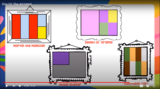Unrestricted Use
CC BY
Rating
0.0 stars

This lesson is Day 12 in a series of 12 lessons around fraction equivalences and comparisons.

Use your math skills to help detectives solve the crime. This video lesson reviews fractions of an area, fractions on a number line, and equivalent fractions. No materials are required.

Subject:
Mathematics
Material Type:
Activity/Lab
Interactive
Lesson
Simulation
Author:
Dawne Coker
06/25/2020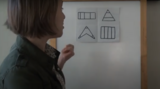Unrestricted Use
Public Domain
Rating
0.0 stars

This lesson is Day 1 in a series of 12 lessons around fraction equivalences and comparisons.

This video lesson encourages students to reason about and visualize fractions. The lesson focuses on the ideas that fractions are fair shares and that fractions can be compared. This lesson assumes that students have some initial experiences with fractions.

Subject:
Mathematics
Material Type:
Interactive
Lesson
Presentation
Simulation
Author:
Dawne Coker
06/25/2020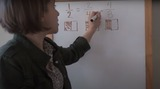Unrestricted Use
Public Domain
Rating
0.0 stars

This lesson is Day 2 in a series of 12 lessons around fraction equivalences and comparisons.

This video lesson uses paper folding to introduce the concept of equivalent fractions. To complete this lesson, make sure you have a square piece of paper (any size) and a crayon

Subject:
Mathematics
Material Type:
Activity/Lab
Interactive
Lesson
Simulation
Author:
Dawne Coker
06/25/2020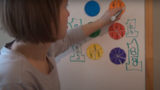Unrestricted Use
CC BY
Rating
0.0 stars

This lesson is Day 2 in a series of 12 lessons around fraction equivalences and comparisons.

This video lesson introduces the idea that fractions can be used to represent 1 whole. To engage in this lesson, students may use virtual circle models from Toy Theater (https://toytheater.com/fraction-circles/)

Subject:
Mathematics
Material Type:
Activity/Lab
Interactive
Lesson
Simulation
Author:
Dawne Coker
06/25/2020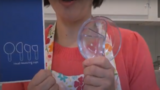Unrestricted Use
CC BY
Rating
0.0 stars

This lesson is Day 4 in a series of 12 lessons around fraction equivalences and comparisons.

This video lesson looks at how we use fractions in the kitchen. Students are challenged to use circle models to find equivalent fractions for a recipe. Students may use virtual circe models from the Toy Theater (https://toytheater.com/fraction-circles/).

Subject:
Mathematics
Material Type:
Activity/Lab
Interactive
Lesson
Simulation
Author:
Dawne Coker
06/25/2020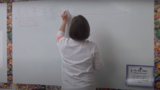Unrestricted Use
CC BY
Rating
0.0 stars

This lesson is Day 9 in a series of 12 lessons around fraction equivalences and comparisons.

This video lesson introduces students to fractions beyond 1 using a choral counting routine. Students connect the fractions to points on a number line and bar models. For this lesson, students need a piece of blank paper and a pencil.

Subject:
Mathematics
Material Type:
Activity/Lab
Interactive
Lesson
Simulation
Author:
Dawne Coker
06/25/2020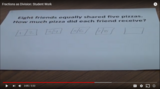Unrestricted Use
CC BY
Rating
0.0 stars

This video shows two different student-generated strategies for solving fair share problems leading to a fraction less than 1. This standard relates to Common Core standard 5.NF.3, and North Carolina standard NC.5.NF.3.

The end of this video helps teachers see how standard NF.3 can be introduced prior to NF.1 (addition/subraction of fractions with unlike denominators).

Subject:
Mathematics
Material Type:
Diagram/Illustration
Reference Material
Teaching/Learning Strategy
Author:
Dawne Coker
06/26/2020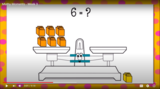Unrestricted Use
CC BY
Rating
0.0 stars

This video lesson examines the meaning of the equal sign as we work to solve unknowns in multiplication and division equations.

Subject:
Mathematics
Material Type:
Activity/Lab
Interactive
Lesson
Simulation
Author:
Dawne Coker
06/26/2020Rating
0.0 stars

The interactive pan balance uses multiple shapes with different values, allowing students to investigate what happens as different shapes are placed on the balance. This student interactive, from Illuminations, provides an interesting environment in which students can consider the concept of equivalence.

Subject:
Geometry
Mathematics
Material Type:
Activity/Lab
Interactive
Lesson Plan
Simulation
Provider:
National Council of Teachers of Mathematics
Author:
Illuminations
02/26/2019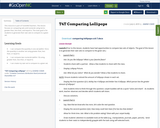Conditional Remix & Share Permitted
CC BY-NC-SA
Rating
0.0 stars

This resource is part of Tools4NCTeachers.

This lesson focuses on comparing sets and numerals using terms greater than, less than, and equal to. The main goal is for students to generate their own sets to compare to a given set.

Subject:
Mathematics
Material Type:
Lesson
Lesson Plan
11/06/2019Unrestricted Use
CC BY
Rating
0.0 stars

This resource is from Tools4NCTeachers.&nbsp;This file contains a set of 6 tasks (including scoring rubric and student recording sheet).&nbsp; The tasks may be&nbsp;used for instruction or assessment.&nbsp; Each task focuses on comparing 3-digit numbers, and using symbols (&gt;,&lt;, =) to make comparisons.Remix this resource to include student work samples or&nbsp;addtional tasks.

Subject:
Mathematics
Material Type:
Activity/Lab
Assessment
Formative Assessment
Author:
DAWNE COKER
06/15/2020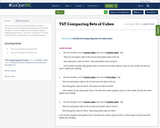Conditional Remix & Share Permitted
CC BY-NC-SA
Rating
0.0 stars

This resource is part of Tools4NCTeachers.

Students compare two sets of objects using matching and counting strategies. The sets of objects are compared using the language "greater than", "less than" or "equal to".

This task may be used for instructional or assessment purposes.

Subject:
Mathematics
Material Type:
Activity/Lab
Assessment
Formative Assessment
11/06/2019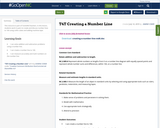Conditional Remix & Share Permitted
CC BY-NC-SA
Rating
0.0 stars

This resource is part of Tools4NCTeachers.

In this lesson, students work in pairs to create and discuss number lines to 100 using unifix cubes and adding machine tape.

Subject:
Mathematics
Material Type:
Lesson
Lesson Plan
11/06/2019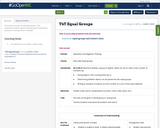Conditional Remix & Share Permitted
CC BY-NC-SA
Rating
0.0 stars

This resource is part of Tools4NCTeachers.

This assessment may be used for instructional or assessment purposes. A scoring rubric is included.

Subject:
Mathematics
Material Type:
Assessment
Formative Assessment
11/06/2019Conditional Remix & Share Permitted
CC BY-NC-SA
Rating
0.0 stars

This resource is part of Tools4NCTeachers.

In this lesson, the students will explore even and odd numbers through a variety of hands-on learning activities. Students will explore numbers up to 100, comparisons of groups, and odd/even relationships of numbers up to 20.

Subject:
Mathematics
Material Type:
Lesson
11/06/2019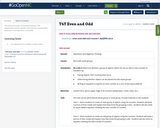Conditional Remix & Share Permitted
CC BY-NC-SA
Rating
0.0 stars

This resource is part of Tools4NCTeachers.

This assessment may be used for instructional or assessment purposes. A scoring rubric is included.

Subject:
Mathematics
Material Type:
Assessment
Formative Assessment